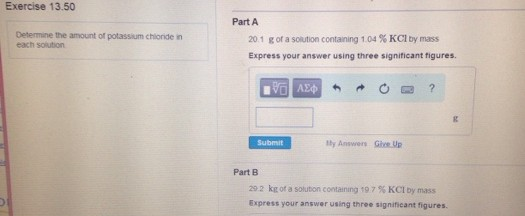# Problem: Determine the amount of potassium chloride in each solution. 20.1 g of a solution containing 1.04 % KCl by mass. Express your answer using three significant figures. 29.2 kg of a solution containing 19.7 % KCl by mass. Express your answer using three significant figures.

🤓 Based on our data, we think this question is relevant for Professor Norton's class at FSCJ.

###### FREE Expert Solution###### Problem Details

Determine the amount of potassium chloride in each solution.

20.1 g of a solution containing 1.04 % KCl by mass. Express your answer using three significant figures.

29.2 kg of a solution containing 19.7 % KCl by mass. Express your answer using three significant figures.